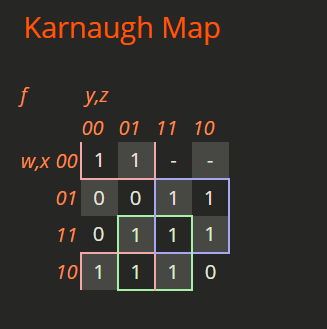# GATE | GATE CS 2021 | Set 2 | Question 62

• Last Updated : 23 May, 2021

Consider a Boolean function f(w,x,y,z) such that

```f(w,0,0,z) = 1
f(1,x,1,z) = x+z
f(w,1,y,z) = wz+y
```

The number of literals in the minimal sum-of-products expression of f is _________ .
(A) 6
(B) 3
(C) 8
(D) 1

Explanation: f(w,0,0,z) = 1
When x = 0, and y = 0, the min-term will be 1 irrespective of w and z.

f(1,x,1,z) = x+z
When w = 1 and y = 1, the min-term will be x or’d with z.

Similarly for the last one.f(w, x, y, z) = sigma(0,1,6,7,8,9,11,13,14,15) + don’t(2,3)
f(w, x, y, z) = x’y’ + wz + xy

Thus, the number of literals will be 6.

Quiz of this Question

My Personal Notes arrow_drop_up
Recommended Articles
Page :